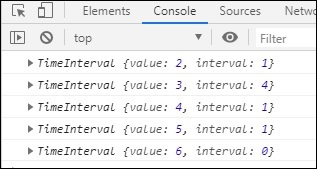# RxJS - Utility Operator timeInterval

This operator will return an object which contains current value and the time elapsed between the current and previous value that is calculated using scheduler input taken.

## Syntax

```timeInterval(scheduler): Observable
```

## Parameters

scheduler(optional) The scheduler input is used to calculate the time elapsed between the current and previous value from source Observable.

## Return value

It will return an observable that will have source values and also the time interval.

## Example

```import { of } from 'rxjs';
import { filter, timeInterval } from 'rxjs/operators';

let list1 = of(2, 3, 4, 5, 6);
let final_val = list1.pipe(timeInterval());
final_val.subscribe(x => console.log(x));
```

## Output